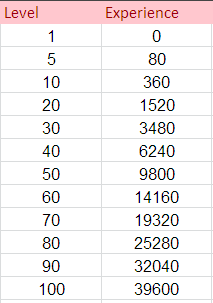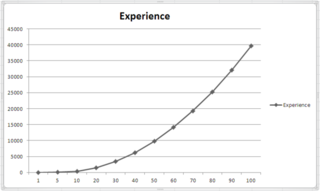# Simple RPG Algorithms

I’m having trouble coming up with attack, defense, etc. curves. I want these all to be interdependent on 4 basic stats. For instance, we have strength, constitution, intelligence, and dexterity. I’m trying to have them all curve up, but I want the values to progress in a nice slow upward way.

I have (x*x+x+3)4 for experience and it results in a pretty nice curve, but the formulas I’m using that are similar to that are giving me garbage for values of health, defense, attack, and damage. How are algorithms like these normally structured?

A google search hasn’t helped me any.Here are some nice algorithms used in FF6, will add a stat growth algo if I can find it.
http://www.rpglegion.com/ff6/ff6alg.txt

Here is some pseudo-code from memory on how I would implement it:

``````var level = 1;

//Default Values - Knight//
var attack = 4;
var defense = 2;

var atkMin = 1.2;
var atkMax = 3.5;

var defMin = 1.1;
var defMax = 2.8;

//You can multiply or add, multply will most likely give you bigger values :D
attack = attack + (level + random(atkMin, atkMax));
defense = defense + (level + random(defMin, defMax ));

//Forgot how to round numbers in Unity :D
attack = round(attack);
defense = round(defense);
``````

Not around a calculator or IDE, so can’t really test it out for myself at the moment.

Though SirVictory’s answer was on point, randoms would just not work for me. I want a constant curve that allows for a continuing variable. Using my original curve, (x*x+x+3)4 I just had to extensively tweak it for the values I wanted. It is important to view certain points that you want the player to progress and be at. A visual of the different values was extremely helpful.These values are accumulated to the whole, so the curve turned out pretty nicely.The only thing I really had to do differently was find the end value I wanted and beginning value I wanted. I was able to accomplish this by scaling the original formula for different values. For instance, dividing it by 30 gave me a nice range for my attack value.

So pretty much, for those of you having trouble finding formulas, find a nice curve that you like and tweak it for each of your other stats.

Credit for the graphs and “pink” table goes to SirVictory.

If you want a more visual control with your curves you can use the animation curve.

Then based off the players currentpoints divided by maxpoints (for stats) or simply plug in your “level” (0.1 = level 10 | 0.5 = level 50 | etc) you can evaluate the curve

float wpnDamage = baseWpnDmg + lvlStrength + (lvlStrengthwpnStrengthModifier) +
lvlSpeed + (lvlSpeed
wpnSpeedModifier) + (baseIncrementValue * (maxLevel/lvlStrength));

e.g: baseWpnDmg = 130; lvlStrength = 10; lvlSpeed = 8; wpnSpeedModifier = 0.6;
wpnStrengthModifier = 0.6; baseIncrementValue = 5; maxLevel = 99;

wpnDamage = 130 + 10 + 6 + 8 + 4.8 + (5 * (99/10)) = 158.8 + 49.5 = 208.3

if we change lvlSpeed and lvlStrength to 25 and 30 respectively:

wpnDamage = 130 + 30 + 18 + 25 + 15 + (5 * (99/30)) = 218 + 16.5 = 234.5

to get a nicer curve you could experiment with changing the baseIncrementValue after a certain number of levels e.g:
if (lvlStrength >= firstLevelCap){
IncrementValue = firstIncrementValue;
} and so on and so on